# How to Count Numbers Nth Digit Equals to Specific Number in Excel

This post will guide you how to count numbers where the Nth digit equal to a specific number in Excel 2013/2016 or Excel office 365. How do I count of numbers in a given range where the Nth digit is equal to a particular number using SUMPRODUCT function and the MID function in Excel.

## General Formula:

The below general formula can help you to count the numbers where the Nth digit equal to a given number in a given range of cells in Excel. Like this:

=SUMPRODUCT(–MID(range,digit,character)=”number”)

## Count Numbers Where The Nth Digit Equal To

Assuming that you have a data of list in range B2:B6, and you want to count numbers where the second digit equals to number “5”, and you can use a formula based on the SUMPRODUCT function and MID function. Like this:

=SUMPRODUCT(–(MID(B2:B6,2,1)=”5″))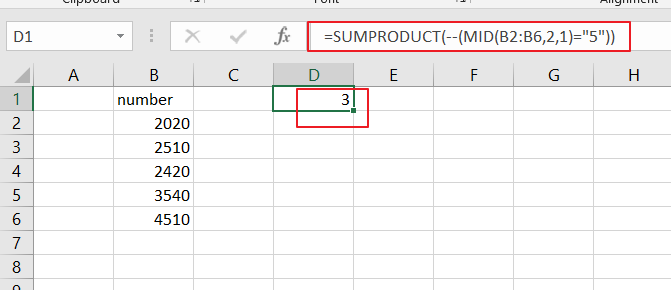Let’s See That How This Formula Works:

= MID(B2:B6,2,1)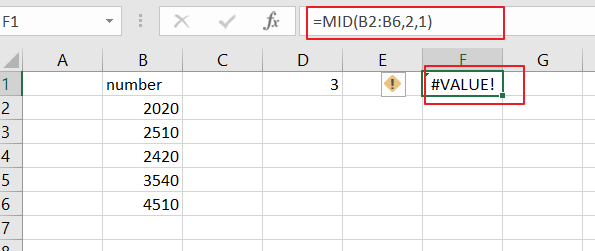The MID function can be used to extract the number or character from the given text string. And if you want to get the second character or number from a given range B2:B6, and you can use the above formula. And the first argument is a cell range, and the second argument specifies the start position, and the third argument indicates that the number of characters you want from the specified position of the text. This formula returns an array result like this:

{“0″;”5″;”4″;”5″;”5”}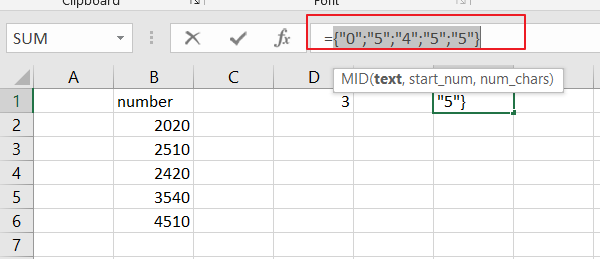This array results contain all the second digit from each cells in the given range B2:B6.

=MID(B2:B6,2,1)=”5″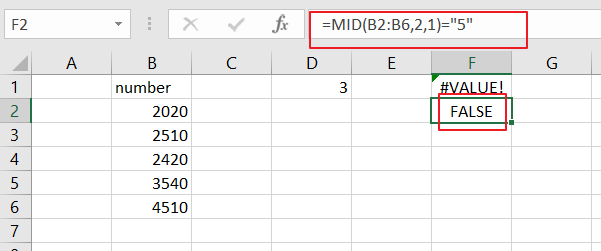The above array result will compare each value to “5” to generate a TRUE or FALSE result. And this formula also returned as an array result of TRUE and FALSE values. Like this:

{FALSE;TRUE;FALSE;TRUE;TRUE}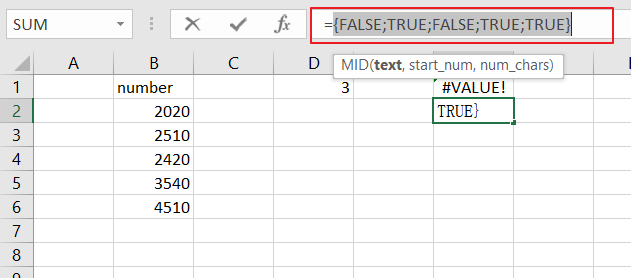=–(MID(B2:B6,2,1)=”5″)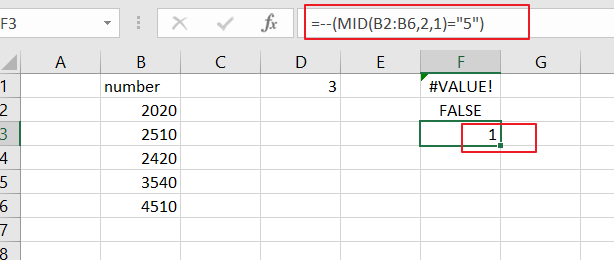Then you need to use a double negative operator to convert TRUE or FALSE values to 1 and 0. It also returned an array result like:

{0;1;0;1;1}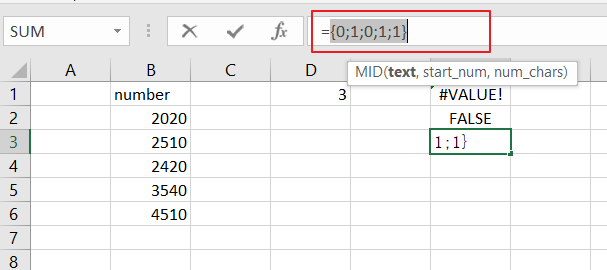SUMPRODUCT function sums the numbers in the above array and returns 3.

### Related Functions

• Excel SUMPRODUCT function
The Excel SUMPRODUCT function multiplies corresponding components in the given one or more arrays or ranges, and returns the sum of those products. The syntax of the SUMPRODUCT function is as below:= SUMPRODUCT (array1,[array2],…)…
• Excel MID function
The Excel MID function returns a substring from a text string at the position that you specify.The syntax of the MID function is as below:= MID (text, start_num, num_chars)…